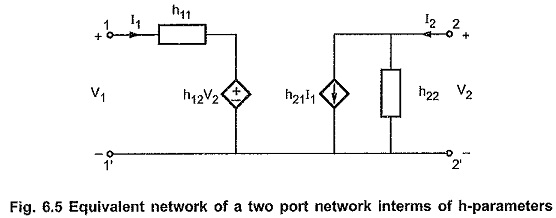## h Parameters of Two Port Network:

h Parameters of Two Port Network are also called hybrid parameters. These parameters are very useful in constructing models for transistors. The transistor parameters cannot be calculated using by either short circuit admittance parameter or open circuit impedance parameter measurement.

These parameters are obtained by expressing voltage at input port and the current at output port in terms of the current at the input port and the voltage at the output port. Thus, the current I1 and voltage V2 are independent variables ; while current I2 and voltage V1 are the dependent variables.

Thus, we have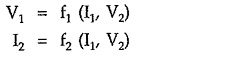In equation form, above relations can be written as,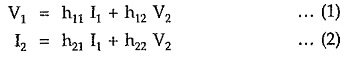In matrix form the above equations can be written as,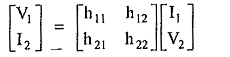The individual h-parameters are defined as follows,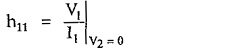This is called short circuit input impedance.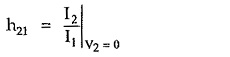This is called forward short circuit current gain.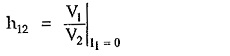This is called reverse open circuit voltage gain.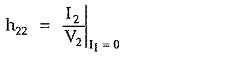This is called open circuit output admittance.

All above parameters are having different units such as ohm for short circuit impedance and mho for open circuit output admittance, the name of the parameter is hybrid parameter.

The equivalent circuit of a two port network interms of hybrid parameters satisfying equations (1) and (2) is as shown in the Fig. 6.5.